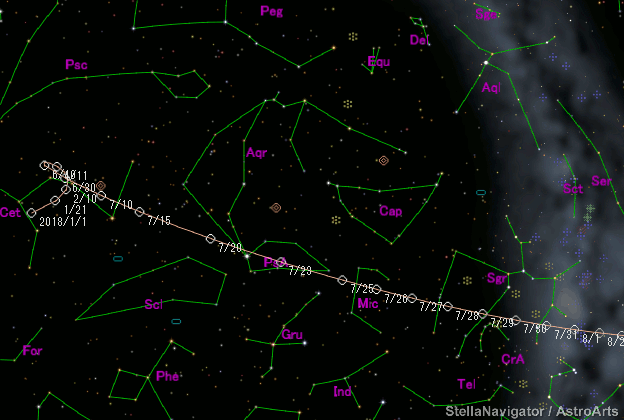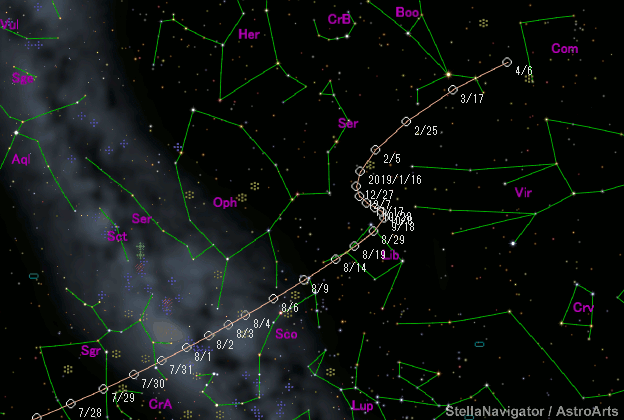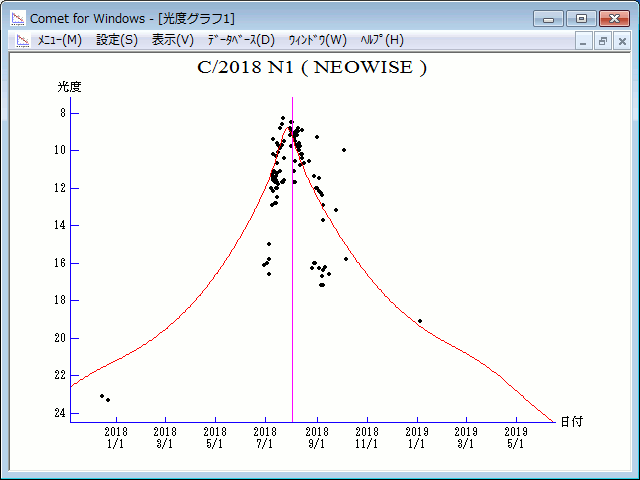\$B%M%*%o%\$%:WB@1(B

C/2018 N1 ( NEOWISE )\$B%W%m%U%#!<%k(B

 \$BH/8+F|(B 2018\$BG/(B7\$B7n(B2\$BF|(B \$BH/8+8wEY(B 16\$BEy(B \$BH/8+ Near-Earth Object Wide-field Infrared Survey Explorer\$B\$B50F;MWAG(B

```Epoch 2018 July 21.0 TT = JDT 2458320.5
T 2018 Aug. 1.98608 TT                                  MPCW
q   1.3073502            (2000.0)            P               Q
z  +0.0029419      Peri.  354.56231     +0.46200652     -0.82535782
+/-0.0002180      Node   292.43988     -0.79988424     -0.54584664
e   0.9961539      Incl.  159.44289     -0.38306028     +0.14434654
From 235 observations 2018 June 29-Oct. 6, mean residual 0".5.
```\$B@1?^(B\$B8wEYJQ2=(B

```        m1 = 9.0 + 5 log\$B&\$(B + 20.0 log r
```\$B50F;MWAG\$O(BM.P.E.C. 2018-U70\$B\$K7G:\\$5\$l\$?\$b\$N\$G\$9!#(B \$B@1?^\$O%9%F%i%J%S%2!<%?(B Ver.10 (\$B%"%9%H%m%"!<%D(B) \$B\$G:n@.\$7\$?\$b\$N\$G\$9!#(B \$B8wEY%0%i%U\$O(BComet for Windows\$B\$G:n@.\$7\$?\$b\$N\$G\$9!#(B